The goal here is to illustrate the “adaptive” nature of the adaptive shrinkage. The shrinkage is adaptive in two senses. First, the amount of shrinkage depends on the distribution $$g$$ of the true effects, which is learned from the data: when $$g$$ is very peaked about zero then ash learns this and deduces that signals should be more strongly shrunk towards zero than when $$g$$ is less peaked about zero. Second, the amount of shrinkage of each observation depends on its standard error: the smaller the standard error, the more informative the data, and so the less shrinkage that occurs. From an Empirical Bayesian perspective both of these points are entirely natural: the posterior depends on both the prior and the likelihood; the prior, $$g$$, is learned from the data, and the likelihood incorporates the standard error of each observation.

First, we load the necessary libraries.

library(ashr)
library(ggplot2)


We simulate from two scenarios: in the first scenario, the effects are more peaked about zero (sim.spiky); in the second scenario, the effects are less peaked at zero (sim.bignormal). A summary of the two data sets is printed at the end of this chunk.

set.seed(100)

# Simulates data sets for experiments below.
rnormmix_datamaker = function (args) {

# generate the proportion of true nulls randomly.
pi0 = runif(1,args$min_pi0,args$max_pi0)
k   = ncomp(args$g) #randomly draw a component comp = sample(1:k,args$nsamp,mixprop(args$g),replace = TRUE) isnull = (runif(args$nsamp,0,1) < pi0)
beta   = ifelse(isnull,0,rnorm(args$nsamp,comp_mean(args$g)[comp],
comp_sd(args$g)[comp])) sebetahat = args$betahatsd
betahat   = beta + rnorm(args$nsamp,0,sebetahat) meta = list(g1 = args$g,beta = beta,pi0 = pi0)
input     = list(betahat = betahat,sebetahat = sebetahat,df = NULL)
return(list(meta = meta,input = input))
}

NSAMP = 1000
s     = 1/rgamma(NSAMP,5,5)

sim.spiky =
rnormmix_datamaker(args = list(g = normalmix(c(0.4,0.2,0.2,0.2),
c(0,0,0,0),
c(0.25,0.5,1,2)),
min_pi0   = 0,
max_pi0   = 0,
nsamp     = NSAMP,
betahatsd = s))

sim.bignormal =
rnormmix_datamaker(args = list(g         = normalmix(1,0,4),
min_pi0   = 0,
max_pi0   = 0,
nsamp     = NSAMP,
betahatsd = s))

cat("Summary of observed beta-hats:\n")
## Summary of observed beta-hats:
print(rbind(spiky     = quantile(sim.spiky$input$betahat,seq(0,1,0.1)),
bignormal = quantile(sim.bignormal$input$betahat,seq(0,1,0.1))),
digits = 3)
##               0%   10%   20%    30%    40%     50%   60%   70%  80%  90% 100%
## spiky      -6.86 -1.99 -1.19 -0.717 -0.326  0.0380 0.385 0.728 1.23 2.04 15.2
## bignormal -14.26 -5.03 -3.48 -1.989 -0.984 -0.0941 1.026 2.141 3.63 5.61 13.4


Now we run ash on both data sets.

beta.spiky.ash     = ash(sim.spiky$input$betahat,s)
beta.bignormal.ash = ash(sim.bignormal$input$betahat,s)


Next we plot the shrunken estimates against the observed values, colored according to the (square root of) precision: precise estimates being colored red, and less precise estimates being blue. Two key features of the plots illustrate the ideas of adaptive shrinkage: i) the estimates under the spiky scenario are shrunk more strongly, illustrating that shrinkage adapts to the underlying distribution of beta; ii) in both cases, estimates with large standard error (blue) are shrunk more than estimates with small standard error (red) illustrating that shrinkage adapts to measurement precision.

make_df_for_ashplot =
function (sim1, sim2, ash1, ash2, name1 = "spiky", name2 = "big-normal") {
n = length(sim1$input$betahat)
x = c(get_lfsr(ash1),get_lfsr(ash2))
return(data.frame(betahat  = c(sim1$input$betahat,sim2$input$betahat),
beta_est = c(get_pm(ash1),get_pm(ash2)),
lfsr     = x,
s        = c(sim1$input$sebetahat,sim2$input$sebetahat),
scenario = c(rep(name1,n),rep(name2,n)),
signif   = x < 0.05))
}

ashplot = function(df,xlab="Observed beta-hat",ylab="Shrunken beta estimate")
ggplot(df,aes(x = betahat,y = beta_est,color = 1/s)) +
xlab(xlab) + ylab(ylab) + geom_point() +
facet_grid(.~scenario) +
geom_abline(intercept = 0,slope = 1,linetype = "dotted") +
mid = "white",high = "red",space = "Lab") +
coord_fixed(ratio = 1)

df = make_df_for_ashplot(sim.spiky,sim.bignormal,beta.spiky.ash,
beta.bignormal.ash)
print(ashplot(df))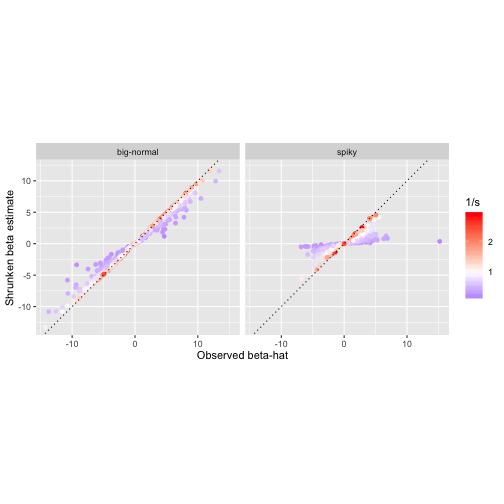A related consequence is that significance of each observation is no longer monotonic with $$p$$ value.

pval_plot = function (df)
ggplot(df,aes(x = pnorm(-abs(betahat/s)),y = lfsr,color = log(s))) +
geom_point() + facet_grid(.~scenario) + xlim(c(0,0.025)) +
xlab("p value") + ylab("lfsr") +
mid = "white",high = "blue")

print(pval_plot(df))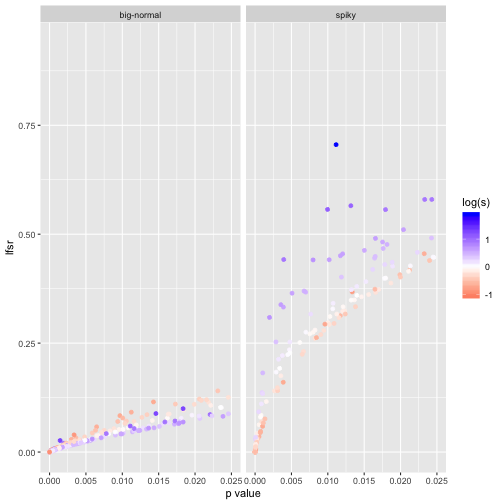Let's see how these are affected by changing the modelling assumptions so that the standardized beta are exchangeable (rather than the beta being exchangeable).

beta.bignormal.ash.ET =
ash(sim.bignormal$input$betahat,s,alpha = 1,mixcompdist = "normal")
beta.spiky.ash.ET =
ash(sim.spiky$input$betahat,s,alpha = 1,mixcompdist = "normal")
df.ET = make_df_for_ashplot(sim.spiky,sim.bignormal,beta.spiky.ash.ET,
beta.bignormal.ash.ET)
ashplot(df.ET,ylab = "Shrunken beta estimate (ET model)")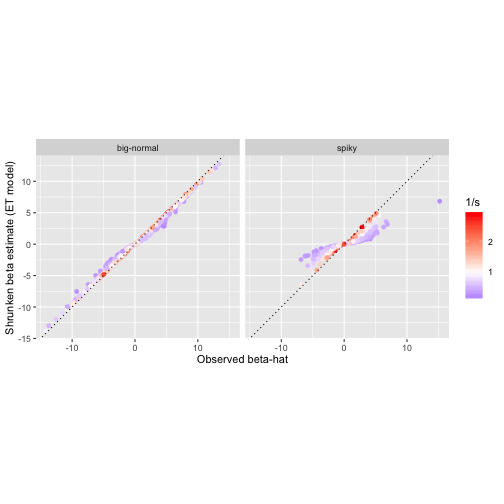pval_plot(df.ET)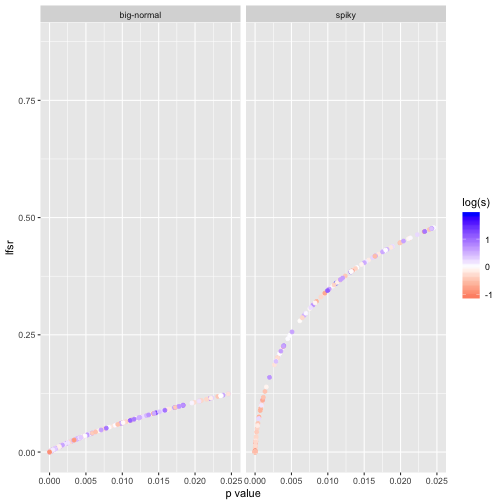This is a “volcano plot” showing effect size against p value. The blue points are “significant” in that they have lfsr < 0.05.

print(ggplot(df,aes(x = betahat,y = -log10(2*pnorm(-abs(betahat/s))),
col = signif)) +
geom_point(alpha = 1,size = 1.75) + facet_grid(.~scenario) +
theme(legend.position = "none") + xlim(c(-10,10)) + ylim(c(0,15)) +
xlab("Effect (beta)") + ylab("-log10 p-value"))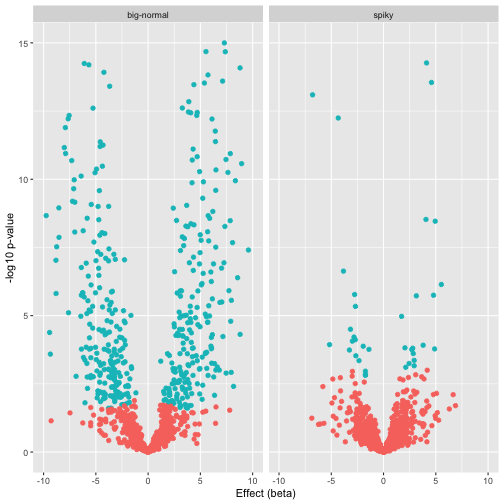In this case the significance by lfsr is not quite the same as cutting off at a given p value (you can see that the decision boundary is not quite the same as drawing a horizontal line), but also not that different, presumably because the standard errors, although varying across observations, do not vary greatly.

## Session information.

print(sessionInfo())

## R version 3.6.2 (2019-12-12)
## Platform: x86_64-apple-darwin15.6.0 (64-bit)
## Running under: macOS Catalina 10.15.3
##
## Matrix products: default
## BLAS:   /Library/Frameworks/R.framework/Versions/3.6/Resources/lib/libRblas.0.dylib
## LAPACK: /Library/Frameworks/R.framework/Versions/3.6/Resources/lib/libRlapack.dylib
##
## locale:
##  en_US.UTF-8/en_US.UTF-8/en_US.UTF-8/C/en_US.UTF-8/en_US.UTF-8
##
## attached base packages:
##  stats     graphics  grDevices utils     datasets  methods   base
##
## other attached packages:
##  ggplot2_3.2.1 ashr_2.2-47
##
## loaded via a namespace (and not attached):
##   Rcpp_1.0.3        highr_0.8         plyr_1.8.5        compiler_3.6.2
##   pillar_1.4.3      tools_3.6.2       digest_0.6.23     evaluate_0.14
##   lifecycle_0.1.0   tibble_2.1.3      gtable_0.3.0      lattice_0.20-38
##  pkgconfig_2.0.3   rlang_0.4.2       Matrix_1.2-18     xfun_0.11
##  invgamma_1.1      withr_2.1.2       stringr_1.4.0     dplyr_0.8.3
##  knitr_1.26        grid_3.6.2        tidyselect_0.2.5  glue_1.3.1
##  R6_2.4.1          mixsqp_0.3-17     irlba_2.3.3       reshape2_1.4.3
##  purrr_0.3.3       farver_2.0.1      magrittr_1.5      scales_1.1.0
##  assertthat_0.2.1  colorspace_1.4-1  labeling_0.3      stringi_1.4.3
##  lazyeval_0.2.2    munsell_0.5.0     truncnorm_1.0-8   SQUAREM_2017.10-1
##  crayon_1.3.4